# Natural Science - Year II

#### Unit 67: Systems and StatesScience Weblecture for Unit 67

 This Unit's Homework Page History Lecture Science Lecture Lab Parents' Notes

# Science Lecture for Unit 67: Systems Science: State Analysis

#### For Class

• Topic area: Information Science
• Terms and concepts to know: State change, iteration, entities, relationships (with respect to databases),the logistics equation, chaos math.
• See historical period(s): Systems Science

Lecture:

## Systems Science

### Systems and States

Systems science uses the idea of "states" throughout its applications. The concept of "states" has its roots in energy studies and thermodynamics. When we study a falling object, we analyze both its kinetic energy and its potential energy. Kinetic energy is an absolute value: it depends on the mass and motions of the object.

But potential energy is always calculated as a change between one PE state and another. We don't calculate the absolute value of potential energy, only the change, ΔPE. In our gravitational fall example, ΔPE is the change in potential energy as the object changes position. If it falls from a height h, then the change in potential energy is the energy it had at the high point compared to the energy at the low point, and this depends only on how far it fell and the force of gravity (F = mg) acting on it:

In systems science, particularly when we use computer modelling to imitate a complex system such as the rotation of a galaxy, we frequently are concerned only with the difference between two states of the system, which must be computed for every single member of the system. Prior to about 1970, studies of multiple-member systems were simply too cumbersome to carry out, but the ability to use not only computers, but multiple computers working together to perform the calculations simultaneously for all the members of the system make studying state changes possible.

In a process over time, systems science uses iteration methods to computer a series of state changes. We begin with an initial state, set up the rules of change, perform a single state change, then using the results of that state change as a new initial state, perform the change again, over and over, for as many cycles necessary to model a given system. We can then rerun the entire simulation with a slightly different set of rules or initial conditions, and see how the outcomes change.

Watch Recreating Our Galaxy in a Supercomputer, a short video from CalTech, describing how astronomers used supercomputer simulations to solve a problem with one model of the formation of the Milky Way Galaxy. (about 3 minutes).

• What are the challenges faced by astronomers in analyzing the formation of a galaxy?
• How many computers were used to perform the simulation?
• What was the problem with the "current model" that the astronomers were trying to resolve?
• What new factor did the astronomers introduce to the model?
• How did this new factor explain our actual observations of the galaxy?

### Database Design

Another type of system we can consider are systems of information. A common way of organizing data using computers uses databases, following the rules originally devised by C. J. Date in the 1960s. A relational database is a kind of state engine, in which we make changes to information at different levels.

To design a database, we start with the things we want to describe somehow, the entities in the real world. We add characteristics to the entities, and then relate different entities by rules. A simple database might involve three entities: a student who is a member of a class group that is itself a particular instance of a course. We can draw a diagram of this information "system" and show the entities and their relationships.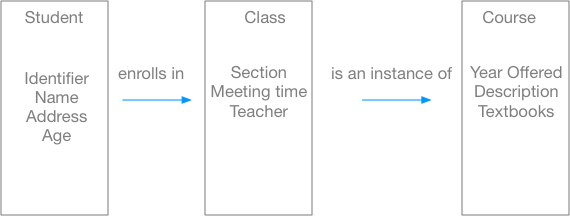To implement this database, we would create a table for the entity "student" with fields for a unique identifier, the student's name, address, age, and other information we might want to keep about the student. We would then link the student with the class in which he enrolls by creating a class table that has information about sections, meeting times, instructors, and other pertinent information. If more than one section of the class is offered, we might want to put this shared information in another table, the "course" table, and only put the information unique to a given instance of the course in a class record.

Analyzing systems this way helps us understand complex relationships between different entities in our system "the school". A student might be a member of more than one section of the same class to resolve conflicts with class meetings times; he might be a member of classes of different courses. The course content and textbooks might change from year to year. Some information might change daily (attendance records!) while other information may be set for a whole year (course descriptions). All of the information at a given instance in time is the "state" of the system. If we make a backup of the database at 3am every night, we can "roll back" our system to an earlier state to recover lost data or fix problems.

### Logistics and Chaos Math

Another outcome of the iterative approach to systems is "chaos math". Bertalanffy's growth equation is an example of an iterative where each state depends on the previous state, but the state itself is open and can be influenced by outside factors. Some situations are still deterministic, that is, we can identify all the factors, but slight variations in conditions produce wildly different results. This is sometimes called the butterfly effect, from the title of a paper given by Edward Lorenz in 1972. Lorenz was using a computer in the early 1970s to study weather phenomena and predictions that used a 12-variables as input. At one point, he decided to check the results of his program output by rerunning the program, but because he didn't want to spend the time doing a detailed analysis, he rounded the variables he had originally to fewer decimal places. In principle, this shouldn't have made a great deal of difference, but he got a completely different prediction. Investigating the mathematics behind his analysis led to a whole new mathematical science, the study of "chaos" situations. Not all mathematical equations result in chaos, but there are a number of iteration situations where a minor change in the starting values creates diversity in the outcome.

A variation of Bertalanffy's growth equation is the logistic equation, usually written this way:

ΔN/Δt is the change in the population of a system over some period of time when we start with N individuals and limit the population to at most K individuals (called the carrying capacity of the system). The value r is the rate of growth. In a population of living things, the rate r might be the birth rate - the death rate, or some combination of similar factors.

We can use a spreadsheet to iterate systems through 15 cycles with different values of r. Here we will start each scenario with 50 individuals as the initial population, and give our environment a carrying capacity of 110 individuals -- that is, the population could double and then some before the ability of the environment to "carry" the population forward is exceeded. The only value we will vary is the rate of growth, r. We'll run the scenario for r = 1, r = 2, r = 3, and r = 3.1.

##### Iteration 1: r = 1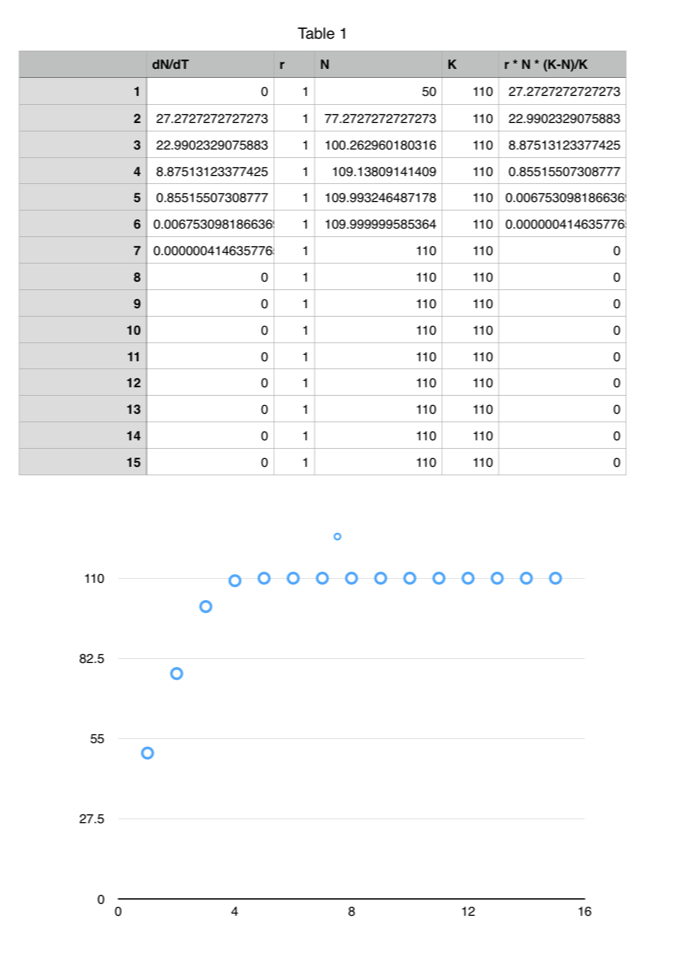In this situation, with the birth + death rate equal to 1, the population grows in seven periods to its carrying capacity and stays there: it has reached a steady state. This result mimics many simple systems, and is similar to exponential growth.

##### Iteration 1: r = 2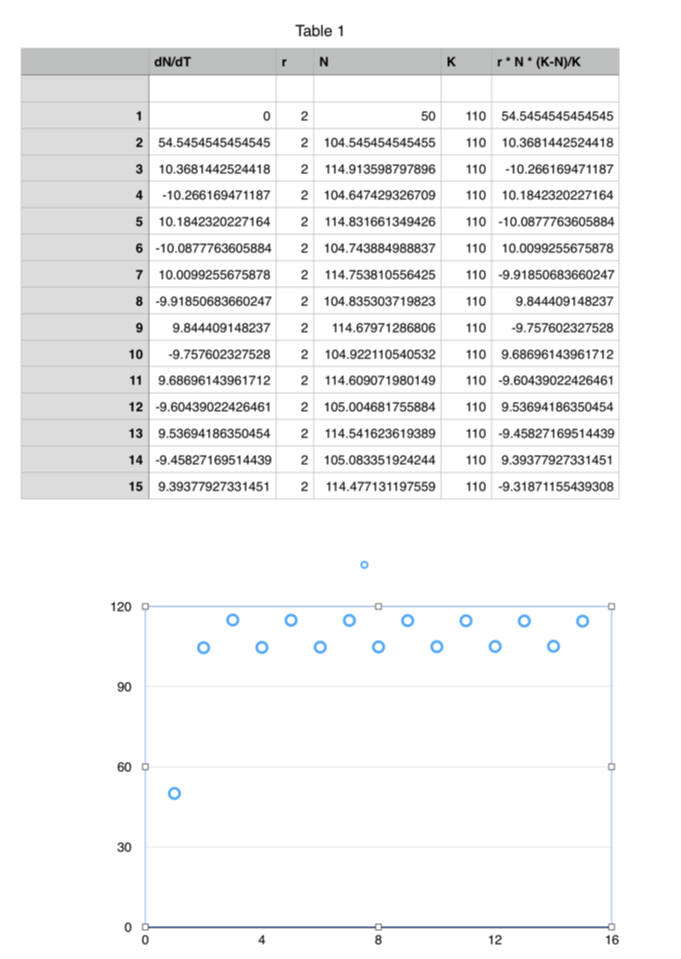With r=2, we get growth to a value near the carrying capacity, but then the population oscillates, either a bit above or a bit below the carrying capacity with each cycle. This situation mimics many feedback systems where the correction kicks in after a limit has been reached, but then the correction overshoots the maximum amount allowed, so that growth recurs until the limit is passed again.

##### Iteration 1: r = 3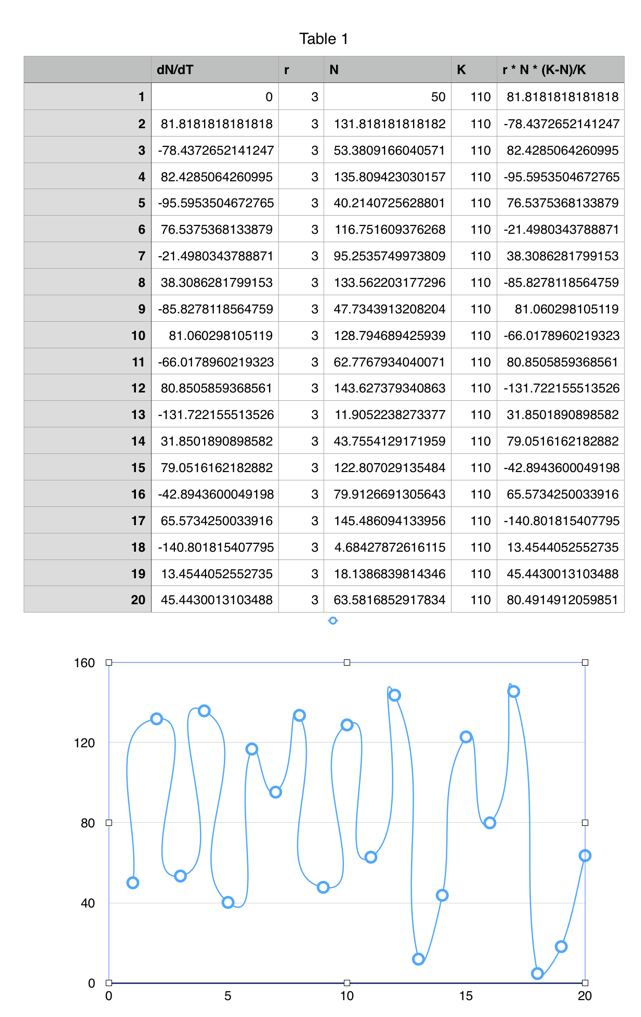With r=3, though, we begint to see a wild variation in growth and decrease. There's no obvious visual pattern to these changes, except that as time goes on, they become more extreme.

##### Iteration 1: r = 3.1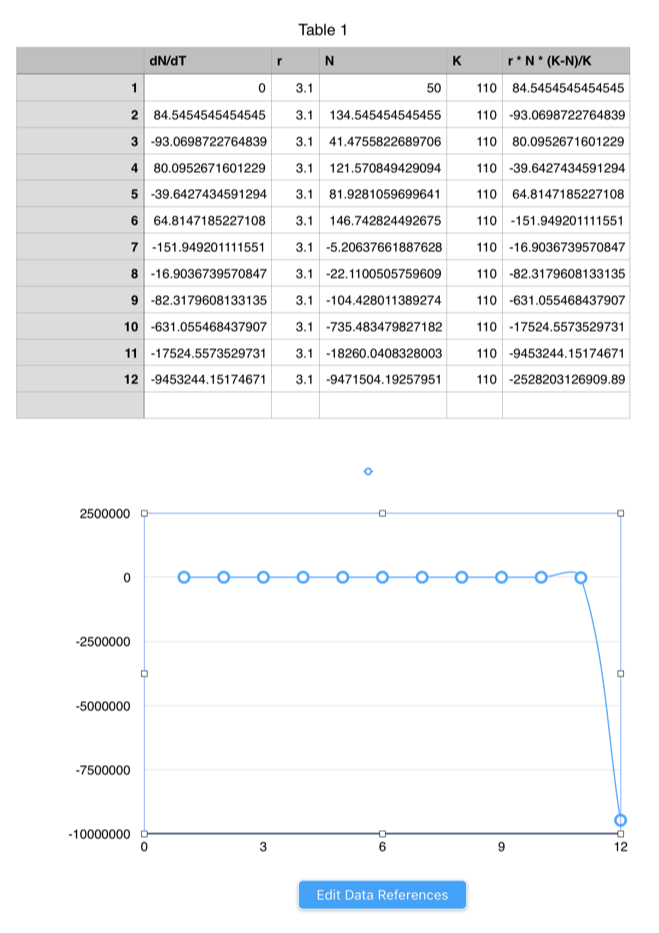With r=3.1, a very small amount of deviation from the previous example, we quickly reach carrying capacity; the system oscillates a bit (that's hard to see on the scale for N), and then it plumets catastrophically.

### Study/Discussion Questions:

• Why was progress using mathematical analysis involving iterative computations slow until around 1970?
• What is an entity? What kinds of relationships can entities in a database have with other entities?
• What is chaos mathematics? How can it be used in simulating natural phenomena?